# Paired Mass Distance(PMD) analysis for GC/LC-MS based non-targeted analysis

## Introduction of Paired Mass Distance analysis

`pmd` package use Paired Mass Distance (PMD) relationship to analysis the GC/LC-MS based non-targeted data. PMD means the distance between two masses or mass to charge ratios. In mass spectrometry, PMD would keep the same value between two masses and two mass to charge ratios(m/z). There are two kinds of PMD involved in this package: PMD from the same compound and PMD from different compounds. In GC/LC-MS or XCMS based non-targeted data analysis, peaks could be separated by chronograph and same compound means ions from similar retention times or ions co-eluted by certain column.

### PMD from the same compound

For MS1 full scan data, we could build retention time(RT) bins to assign peaks into different RT groups by retention time hierarchical clustering analysis. For each RT group, the peaks should come from same compounds or co-elutes. If certain PMD appeared in multiple RT groups, it would be related to the relationship about adducts, neutral loss, isotopologues or common fragments ions.

### PMD from different compounds

The peaks from different retention time groups would like to be different compounds separated by chronograph. The PMD would reflect the relationship about homologous series or chemical reactions.

GlobalStd algorithm use the PMD within same RT group to find independent peaks among certain data set. Then, structure/reaction directed analysis use PMD from different RT groups to screen important compounds or reactions.

## Data format

The input data should be a `list` object with at least two elements from a peaks list:

• mass to charge ratio with name of `mz`, high resolution mass spectrometry is required
• retention time with name of `rt`

However, I suggested to add intensity and group information to the list for validation of PMD analysis.

In this package, a data set from in vivo solid phase micro-extraction(SPME) was attached. This data set contain 9 samples from 3 fish with triplicates samples for each fish. Here is the data structure:

``````library(pmd)
data("spmeinvivo")
str(spmeinvivo)
#> List of 4
#>  \$ data : num [1:1459, 1:9] 1095 10439 10154 2797 90211 ...
#>   ..- attr(*, "dimnames")=List of 2
#>   .. ..\$ : chr [1:1459] "100.1/170" "100.5/86" "101/85" "103.1/348" ...
#>   .. ..\$ : chr [1:9] "1405_Fish1_F1" "1405_Fish1_F2" "1405_Fish1_F3" "1405_Fish2_F1" ...
#>  \$ group:'data.frame':   9 obs. of  2 variables:
#>   ..\$ sample_name : chr [1:9] "1405_Fish1_F1" "1405_Fish1_F2" "1405_Fish1_F3" "1405_Fish2_F1" ...
#>   ..\$ sample_group: chr [1:9] "fish1" "fish1" "fish1" "fish2" ...
#>  \$ mz   : num [1:1459] 100 101 101 103 104 ...
#>  \$ rt   : num [1:1459] 170.2 86.3 84.9 348.1 48.8 ...``````

You could build this `list` or `mzrt` object from the `xcms` objects via `enviGCMS` package. When you have a `xcmsSet` object or `XCMSnExp` object named `xset`, you could use `enviGCMS::getmzrt(xset)` to get such list. Of course you could build such list by yourself.

## GlobalStd algorithm

GlobalStd algorithm try to find independent peaks among certain peaks list. The first step is retention time hierarchical clustering analysis. The second step is to find the relationship among adducts, neutral loss, isotopologues and common fragments ions. The third step is to screen the independent peaks.

Here is a workflow for this algorithm:

``knitr::include_graphics('https://yufree.github.io/presentation/figure/GlobalStd.png')``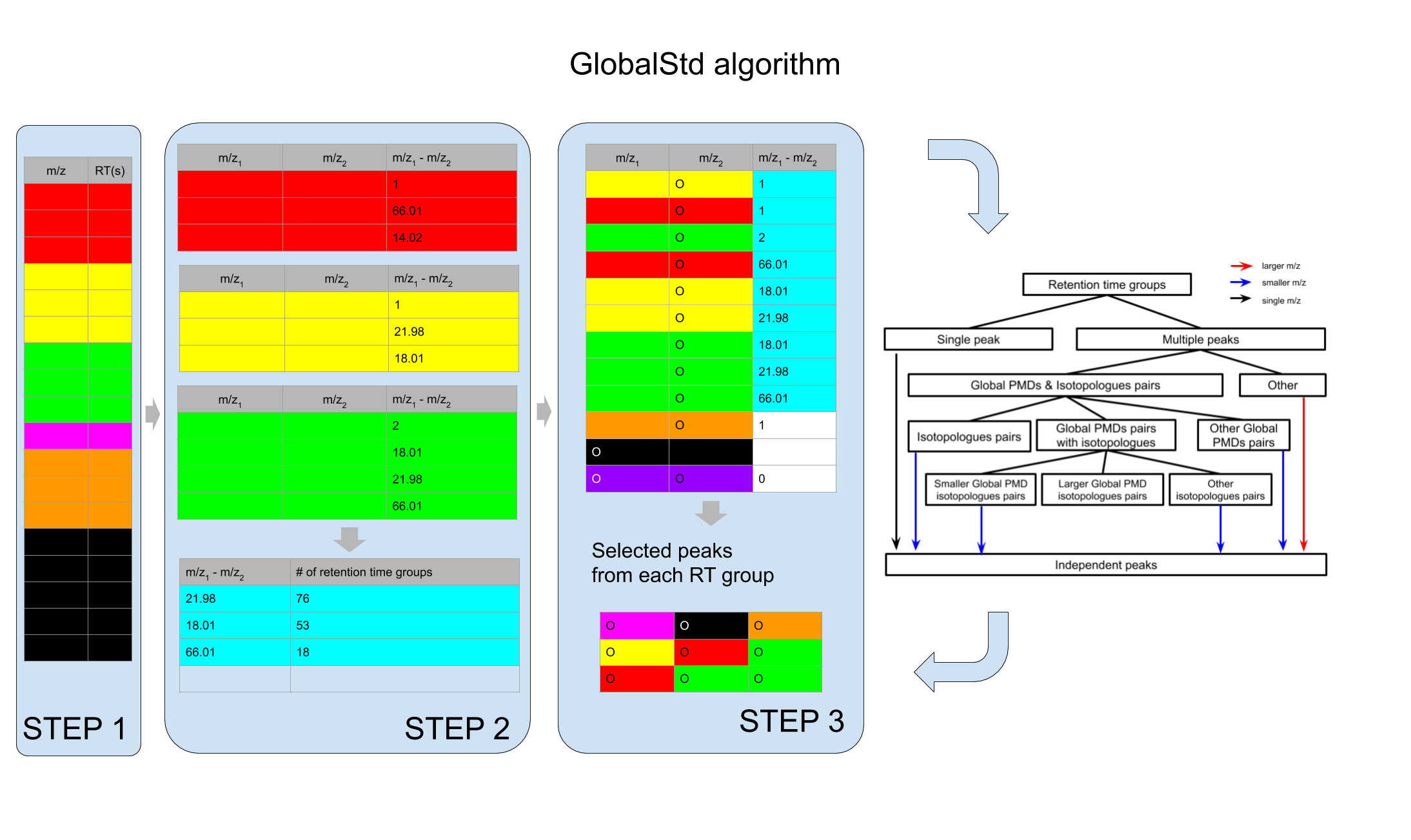### STEP1: Retention time hierarchical clustering

``````pmd <- getpaired(spmeinvivo)
#> 75 retention time cluster found.
#> 369 paired masses found
#> 5 unique within RT clusters high frequency PMD(s) used for further investigation.
#> The unique within RT clusters high frequency PMD(s) is(are)  28.03 21.98 44.03 17.03 18.01.
#> 719 isotopologue(s) related paired mass found.
#> 492 multi-charger(s) related paired mass found.
plotrtg(pmd)``````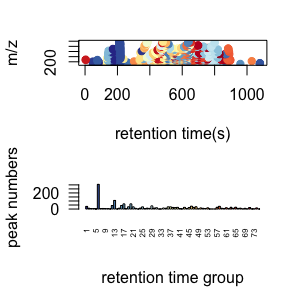This plot would show the distribution of RT groups. The `rtcutoff` in function `getpaired` could be used to set the cutoff of the distances in retention time hierarchical clustering analysis. Retention time cluster cutoff should fit the peak picking algorithm. For HPLC, 10 is suggested and 5 could be used for UPLC.

Global PMD’s retention time group numbers should be around 20 percent of the retention time cluster numbers. For example, if you find 100 retention time clusters, I suggested you use 20 as the cutoff of empirical global PMD’s retention time group numbers. If you don’t specificlly assign a value to `ng`, the algorithm will select such recommendation by default setting.

Take care of the retention time cluster with lots of peaks. In this case, such cluster could be co-eluted compounds on certain column. It would be wise to trim the retention time window for high quality peaks. Another important hint is that pre-filter your peak list by black samples or other quality control samples. Otherwise the running time would be long and lots of pmd relationship would be just from noise.

### STEP2: Relationship among adducts, neutral loss, isotopologues and common fragments ions

The `ng` in function `getpaired` could be used to set cutoff of global PMD’s retention time group numbers. If `ng` is 10, at least 10 of the retention time groups should contain the shown PMD relationship. You could use `plotpaired` to show the distribution.

``plotpaired(pmd)``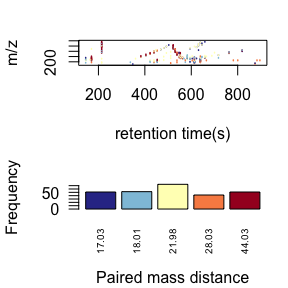You could also show the distribution of PMD relationship by index:

``````# show the unique PMD found by getpaired function
for(i in 1:length(unique(pmd\$paired\$diff2))){
diff <- unique(pmd\$paired\$diff2)[i]
index <- pmd\$paired\$diff2 == diff
plotpaired(pmd,index)
}``````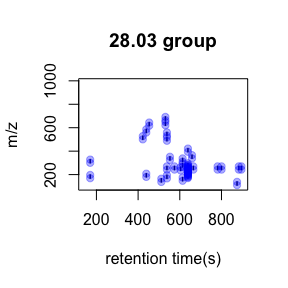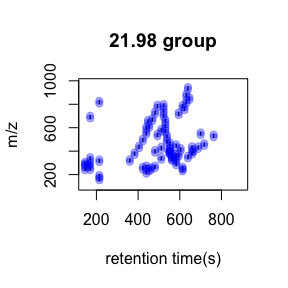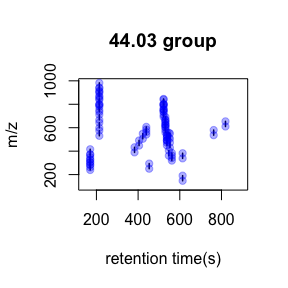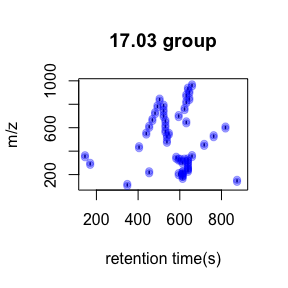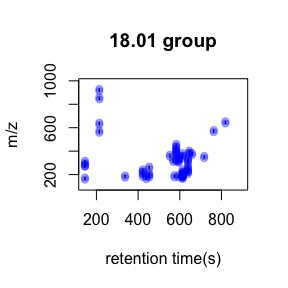This is an easy way to find potential adducts of the data by high frequency PMD from the same compound. For example, 21.98 Da could be the mass distances between \([M+H]^+\) and \([M+Na]^+\). In this case, user could find the potential adducts or neutral loss even when they have no preferred adducts list. If one adduct exist in certain analytical system, the high frequency PMD will reveal such relationship. The high frequency PMD list could also be used to check the fragmental pattern of in-source reactions as long as such patterns are popular among all collected ions.

### STEP3: Screen the independent peaks

You could use `getstd` function to get the independent peaks. Independent peaks mean the peaks list removing the redundant peaks such as adducts, neutral loss, isotopologues and comment fragments ions found by PMD analysis in STEP2. Ideally, those peaks could be molecular ions while they might still contain redundant peaks.

``````std <- getstd(pmd)
#> 8 retention group(s) have single peaks. 14 23 32 33 54 55 56 75
#> 11 group(s) with multiple peaks while no isotope/paired relationship 4 5 7 8 11 41 42 49 68 72 73
#> 9 group(s) with multiple peaks with isotope without paired relationship 2 9 22 26 52 62 64 66 70
#> 4 group(s) with paired relationship without isotope 1 10 15 18
#> 43 group(s) with paired relationship and isotope 3 6 12 13 16 17 19 20 21 24 25 27 28 29 30 31 34 35 36 37 38 39 40 43 44 45 46 47 48 50 51 53 57 58 59 60 61 63 65 67 69 71 74
#> 291 std mass found.``````

Here you could plot the peaks by `plotstd` function to show the distribution of independent peaks:

``plotstd(std)``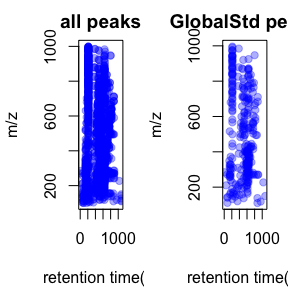You could also plot the peaks distribution by assign a retention time group via `plotstdrt`:

``````par(mfrow = c(2,3))
plotstdrt(std,rtcluster = 23,main = 'Retention time group 23')
plotstdrt(std,rtcluster = 9,main = 'Retention time group 9')
plotstdrt(std,rtcluster = 18,main = 'Retention time group 18')
plotstdrt(std,rtcluster = 67,main = 'Retention time group 67')
plotstdrt(std,rtcluster = 49,main = 'Retention time group 49')
plotstdrt(std,rtcluster = 6,main = 'Retention time group 6')``````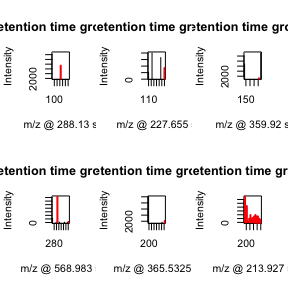### Extra filter with correlation coefficient cutoff

Original GlobalStd algorithm only use mass to charge ratio and retention time of peaks to select independent peaks. However, if intensity data across samples are available, correlation coefficient of paired ions could be used to further filter the random noise in high frequency PMDs. You could set up cutoff of Pearson Correlation Coefficient between peaks to refine the peaks selected by GlobalStd within same retention time groups. In this case, the numbers of selected independent peaks will be further reduced. When you use this parameter, make sure the intensity data are from real samples instead of blank samples, which will affect the calculation of correlation coefficient.

``````std2 <- getstd(pmd,corcutoff = 0.9)
#> 8 retention group(s) have single peaks. 14 23 32 33 54 55 56 75
#> 23 group(s) with multiple peaks while no isotope/paired relationship 2 4 5 7 8 10 11 15 18 26 35 39 41 42 49 50 59 62 68 69 70 72 73
#> 14 group(s) with multiple peaks with isotope without paired relationship 9 12 22 24 27 28 34 51 52 57 60 64 66 71
#> 3 group(s) with paired relationship without isotope 1 53 74
#> 27 group(s) with paired relationship and isotope 3 6 13 16 17 19 20 21 25 29 30 31 36 37 38 40 43 44 45 46 47 48 58 61 63 65 67
#> 120 std mass found.``````

## Validation by principal components analysis(PCA)

You need to check the GlobalStd algorithm’s results by principal components analysis(PCA). If we removed too much peaks containing information, the score plot of reduced data set would show great changes.

``````library(enviGCMS)
par(mfrow = c(2,2),mar = c(4,4,2,1)+0.1)
plotpca(std\$data,lv = as.numeric(as.factor(std\$group\$sample_group)),main = "all peaks")
plotpca(std\$data[std\$stdmassindex,],lv = as.numeric(as.factor(std\$group\$sample_group)),main = paste(sum(std\$stdmassindex),"independent peaks"))
plotpca(std2\$data[std2\$stdmassindex,],lv = as.numeric(as.factor(std\$group\$sample_group)),main = paste(sum(std2\$stdmassindex),"reduced independent peaks"))``````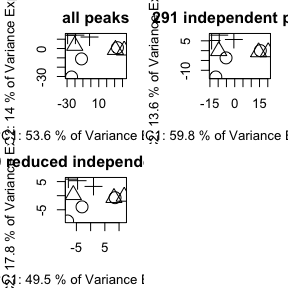You might find original GlobalStd algorithm show a similar PCA score plot with original data while GlobalStd algorithm considering intensity data seems change the profile. The major reason is that correlation coefficient option in the algorithm will remove the paired ions without strong correlation. It will be aggressive to remove low intensity peaks, which are vulnerable by baseline noise. However, such options would be helpful if you only concern high quanlity peaks for following analysis. Otherwise, original GlobalStd will keep the most information for explorer purpose.

## Comparison with other pseudo spectra extraction method

GlobalStd algorithm in `pmd` package could be treated as a method to extract pseudo spectra. You could use `getcluster` to get peaks groups information for all GlobalStd peaks. This function would consider the merge of GlobalStd peaks when certain peak is involved in multiple clusters. Then you could choose export peaks with the highest intensities or base peaks in each GlobalStd merged peaks groups. Meanwhile, you could also include the correlation coefficient cutoff to further improve the data quality.

``````stdcluster <- getcluster(std)
# extract pseudospectra for std peak 71
idx <- unique(stdcluster\$cluster\$largei[stdcluster\$cluster\$i==71])
plot(stdcluster\$cluster\$mz[stdcluster\$cluster\$largei==idx],stdcluster\$cluster\$ins[stdcluster\$cluster\$largei==idx],type = 'h',xlab = 'm/z',ylab = 'intensity',main = 'pseudo spectra for GlobalStd peak 71')``````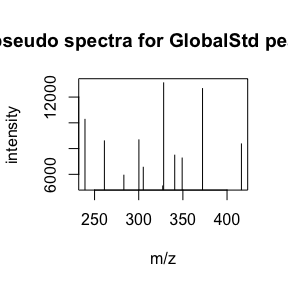``````# export peaks with the highest intensities in each GlobalStd peaks groups.
data <- stdcluster\$data[stdcluster\$stdmassindex2,]
# considering the correlation coefficient cutoff
stdcluster2 <- getcluster(std, corcutoff = 0.9)
# considering the correlation coefficient cutoff for both psedospectra extraction and GlobalStd algorithm
stdcluster3 <- getcluster(std2, corcutoff = 0.9)``````

We supplied `getcorcluster` to find peaks groups by correlation analysis only. The base peaks of correlation cluster were selected to stand for the compounds.

``````corcluster <- getcorcluster(spmeinvivo)
#> 75 retention time cluster found.
# extract pseudospectra 1@46
peak <- corcluster\$cluster[corcluster\$cluster\$largei == '1@46',]
plot(peak\$ins~peak\$mz,type = 'h',xlab = 'm/z',ylab = 'intensity',main = 'pseudo spectra for correlation cluster')``````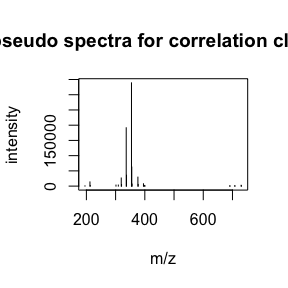Then we could compare the compare reduced result using PCA similarity factor. A good peak selection algorithm could show a high PCA similarity factor compared with original data set while retain the minimized number of peaks.

``````par(mfrow = c(3,3),mar = c(4,4,2,1)+0.1)
plotpca(std\$data[std\$stdmassindex,],lv = as.numeric(as.factor(std\$group\$sample_group)),main = paste(sum(std\$stdmassindex),"independent peaks"))
plotpca(std\$data[stdcluster\$stdmassindex2,],lv = as.numeric(as.factor(std\$group\$sample_group)),main = paste(sum(stdcluster\$stdmassindex2),"independent base peaks"))
plotpca(std\$data[stdcluster2\$stdmassindex2,],lv = as.numeric(as.factor(std\$group\$sample_group)),main = paste(sum(stdcluster2\$stdmassindex2),"independent reduced base peaks"))
plotpca(std\$data[corcluster\$stdmassindex,],lv = as.numeric(as.factor(std\$group\$sample_group)),main = paste(sum(corcluster\$stdmassindex),"peaks without correlationship"))
plotpca(std\$data[corcluster\$stdmassindex2,],lv = as.numeric(as.factor(std\$group\$sample_group)),main = paste(sum(corcluster\$stdmassindex2),"base peaks without correlationship"))
plotpca(std\$data,lv = as.numeric(as.factor(std\$group\$sample_group)),main = paste(nrow(std\$data),"all peaks"))
plotpca(std\$data[stdcluster3\$stdmassindex2,],lv = as.numeric(as.factor(std\$group\$sample_group)),main = paste(sum(stdcluster3\$stdmassindex2),"reduced independent base peaks"))
pcasf(std\$data, std\$data[std\$stdmassindex,])
#>     pcasf
#> 0.9993497
pcasf(std\$data, std\$data[stdcluster\$stdmassindex2,])
#>     pcasf
#> 0.9993578
pcasf(std\$data, std\$data[stdcluster2\$stdmassindex2,])
#>    pcasf
#> 0.999346
pcasf(std\$data, std\$data[corcluster\$stdmassindex,])
#>     pcasf
#> 0.9471586
pcasf(std\$data, std\$data[corcluster\$stdmassindex2,])
#>     pcasf
#> 0.9497193
pcasf(std\$data, std\$data[stdcluster3\$stdmassindex2,])
#>    pcasf
#> 0.713527``````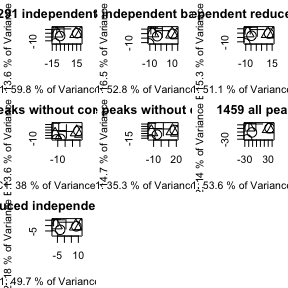In this case, five peaks selection algorithms are fine to stand for the original peaks with PCA similarity score larger than 0.9. However, the independent base peaks retain the most information with relative low numbers of peaks.

## Structure/Reaction directed analysis

`getsda` function could be used to perform Structure/reaction directed analysis. The cutoff of frequency is automate found by PMD network analysis with the largest mean distance of all nodes.

``````sda <- getsda(std)
#> PMD frequency cutoff is 6 by PMD network analysis with largest network average distance 6.67 .
#> 53 groups were found as high frequency PMD group.
#> 0 was found as high frequency PMD.
#> 1.98 was found as high frequency PMD.
#> 2.01 was found as high frequency PMD.
#> 2.02 was found as high frequency PMD.
#> 6.97 was found as high frequency PMD.
#> 11.96 was found as high frequency PMD.
#> 12 was found as high frequency PMD.
#> 13.98 was found as high frequency PMD.
#> 14.02 was found as high frequency PMD.
#> 14.05 was found as high frequency PMD.
#> 15.99 was found as high frequency PMD.
#> 16.03 was found as high frequency PMD.
#> 19.04 was found as high frequency PMD.
#> 28.03 was found as high frequency PMD.
#> 30.05 was found as high frequency PMD.
#> 31.99 was found as high frequency PMD.
#> 33.02 was found as high frequency PMD.
#> 37.02 was found as high frequency PMD.
#> 42.05 was found as high frequency PMD.
#> 48.04 was found as high frequency PMD.
#> 48.98 was found as high frequency PMD.
#> 49.02 was found as high frequency PMD.
#> 54.05 was found as high frequency PMD.
#> 56.06 was found as high frequency PMD.
#> 56.1 was found as high frequency PMD.
#> 58.04 was found as high frequency PMD.
#> 58.08 was found as high frequency PMD.
#> 58.11 was found as high frequency PMD.
#> 63.96 was found as high frequency PMD.
#> 66.05 was found as high frequency PMD.
#> 68.06 was found as high frequency PMD.
#> 70.04 was found as high frequency PMD.
#> 70.08 was found as high frequency PMD.
#> 74.02 was found as high frequency PMD.
#> 80.03 was found as high frequency PMD.
#> 82.08 was found as high frequency PMD.
#> 88.05 was found as high frequency PMD.
#> 91.1 was found as high frequency PMD.
#> 93.12 was found as high frequency PMD.
#> 94.1 was found as high frequency PMD.
#> 96.09 was found as high frequency PMD.
#> 101.05 was found as high frequency PMD.
#> 108.13 was found as high frequency PMD.
#> 110.11 was found as high frequency PMD.
#> 112.16 was found as high frequency PMD.
#> 116.08 was found as high frequency PMD.
#> 122.15 was found as high frequency PMD.
#> 124.16 was found as high frequency PMD.
#> 126.14 was found as high frequency PMD.
#> 144.18 was found as high frequency PMD.
#> 148.04 was found as high frequency PMD.
#> 150.2 was found as high frequency PMD.
#> 173.18 was found as high frequency PMD.``````

Such largest mean distance of all nodes is calculated for top 1 to 100 (if possible) high frequency PMDs. Here is a demo for the network generation process.

``````library(igraph)
#>
#> Attaching package: 'igraph'
#> The following objects are masked from 'package:stats':
#>
#>     decompose, spectrum
#> The following object is masked from 'package:base':
#>
#>     union
cdf <- sda\$sda
# get the PMDs and frequency
pmds <- as.numeric(names(sort(table(cdf\$diff2),decreasing = T)))
freq <- sort(table(cdf\$diff2),decreasing = T)
# filter the frequency larger than 10 for demo
pmds <- pmds[freq>10]
cdf <- sda\$sda[sda\$sda\$diff2 %in% pmds,]
g <- igraph::graph_from_data_frame(cdf,directed = F)
l <- igraph::layout_with_fr(g)
for(i in 1:length(pmds)){
g2 <- igraph::delete_edges(g,which(E(g)\$diff2%in%pmds[1:i]))
plot(g2,edge.width=1,vertex.label="",vertex.size=1,layout=l,main=paste('Top',length(pmds)-i,'high frequency PMDs'))
}``````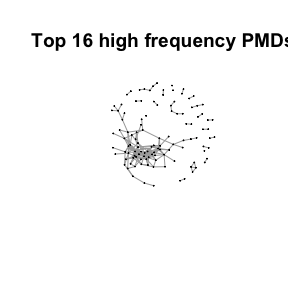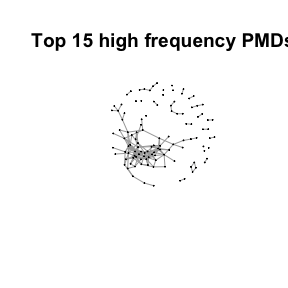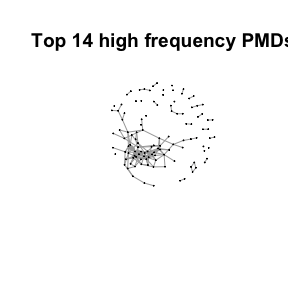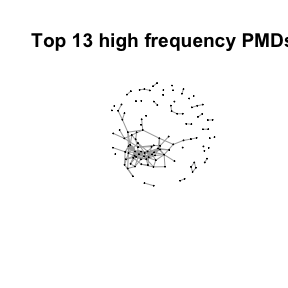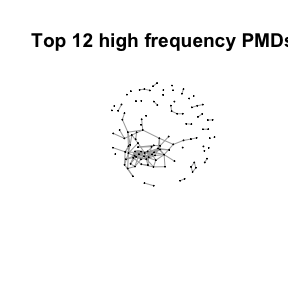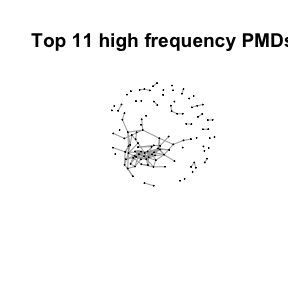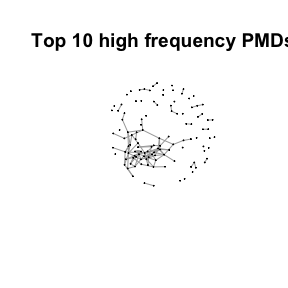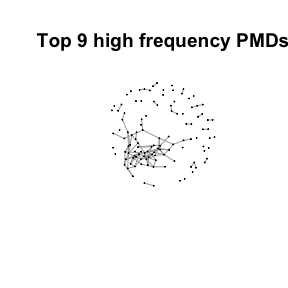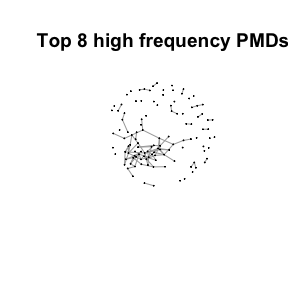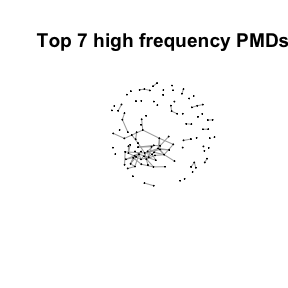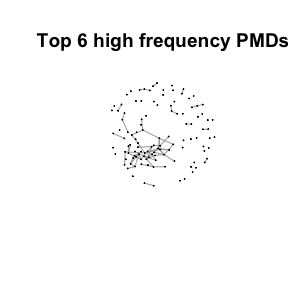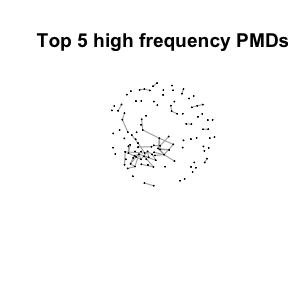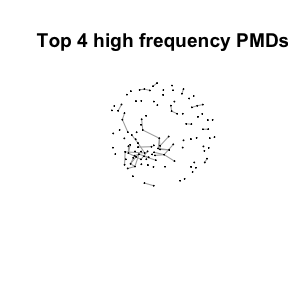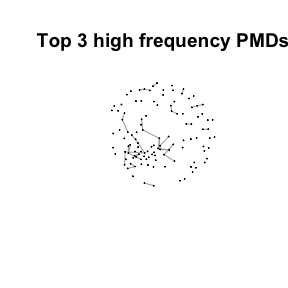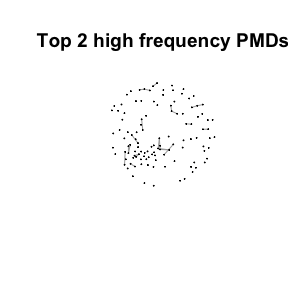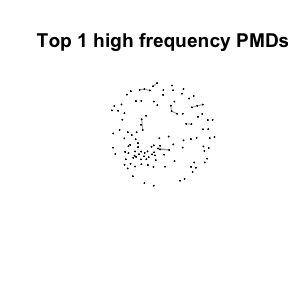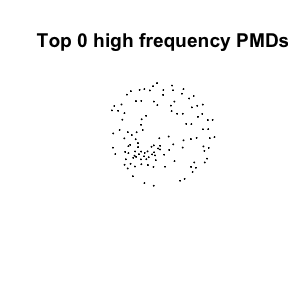Here we could find more and more compounds will be connected with more high frequency PMDs. Meanwhile, the mean distance of all network nodes will increase. However, some PMDs are generated by random combination of ions. In this case, if we included those PMDs for the network, the mean distance of all network nodes will decrease. Here, the largest mean distance means no more information will be found for certain data set and such value is used as the cutoff for high frequency PMDs selection.

You could use `plotstdsda` to show the distribution of the selected paired peaks.

``plotstdsda(sda)``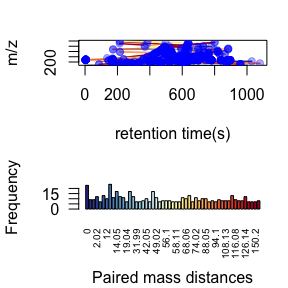You could also use index to show the distribution of certain PMDs.

``````par(mfrow = c(1,3),mar = c(4,4,2,1)+0.1)
plotstdsda(sda,sda\$sda\$diff2 == 2.02)
plotstdsda(sda,sda\$sda\$diff2 == 28.03)
plotstdsda(sda,sda\$sda\$diff2 == 58.04)``````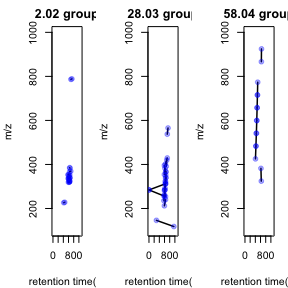Structure/reaction directed analysis could be directly performed on all the peaks, which is slow to process:

``````sdaall <- getsda(spmeinvivo)
#> PMD frequency cutoff is 104 by PMD network analysis with largest network average distance 14.06 .
#> 6 groups were found as high frequency PMD group.
#> 0 was found as high frequency PMD.
#> 2.02 was found as high frequency PMD.
#> 28.03 was found as high frequency PMD.
#> 31.01 was found as high frequency PMD.
#> 58.04 was found as high frequency PMD.
#> 116.08 was found as high frequency PMD.
par(mfrow = c(1,3),mar = c(4,4,2,1)+0.1)
plotstdsda(sdaall,sdaall\$sda\$diff2 == 2.02)
plotstdsda(sdaall,sdaall\$sda\$diff2 == 28.03)
plotstdsda(sdaall,sdaall\$sda\$diff2 == 58.04)``````